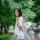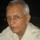Brainy Puzzles
Puzzle Solvers: Interesting brainy puzzles shared by fellow puzzlers
406 Members
Join this group to post and comment.Ankita Katdare • Sep 7, 2018

# Dropping Eggs Puzzle For 2 Eggs and 100 Floors: Correct Answer Anyone?

A friend asked me this question, the other day -

There is a building of 100 floors  If an egg drops from the Nth floor or above it will break. If it’s dropped from any floor below, it will not break.

You’re given 2 eggs. Find N, while minimizing the number of drops for the worst case.

Also, what will be the answer when an Infinite Number of eggs are given?

Quite mind boggling this puzzle is! ð®Rashmi Gandhi • Jun 25, 2019

Wow this is a tough one! I am going to try coming up with a solution right now. Working this thing up on paper..Prudvi Raj • Jul 10, 2019

Can u explain these lines further"

Find N, while minimizing the number of drops for the worst case.

Also, what will be the answer when an Infinite Number of eggs are given?"Prudvi Raj • Jul 10, 2019

I think the question is inadequateIf two eggs are given, the worst case minimum number of drops will be higher if N is odd.

The number of drops needed in this case will be 1+((N+1)/2).

If N is even, then the number of drops will be 1+(N/2).If  the number of eggs is unlimited (not infinity!), then it appears that 7 drops should identify N.To check the validity of 7 drops, I generated 10 random numbers between 1 and 100 and checked that all of them could be achieved with 7 drops.Apply Divide and conquer strategy